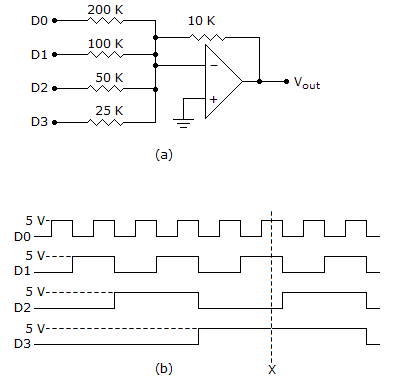# Digital Electronics - Interfacing to the Analog World

### Exercise :: Interfacing to the Analog World - Filling the Blanks

1.

There are many applications in which analog data must be digitized and transferred into a computer's memory. The process by which the computer acquires these digitized analog data is referred to as ________.

 A. sampling B. multiplexing C. data acquisition D. prescaling

Explanation:

No answer description available for this question. Let us discuss.

2.

When analog inputs from several sources are to be converted, a(n) ________ technique can be used.

 A. demultiplexing B. multiplexing C. R/2R D. comparator

Explanation:

No answer description available for this question. Let us discuss.

3.

The output of the circuit in the given figure (a) at point X on figure (b) will be ________.A. 1.011 V B. 2.75 V C. –1.011 V D. –2.75 V

Explanation:

No answer description available for this question. Let us discuss.

4.

The stability of the ADC process can be improved by using a(n) ________ to hold the analog voltage constant while the A/D conversion is taking place.

 A. sample-and-hold circuit B. op-amp comparator C. NPN amp D. current loop

Explanation:

No answer description available for this question. Let us discuss.

5.

The characteristic that a change of one binary step on the input of a DAC should cause exactly one step change on the output is called ________.

 A. resolution B. linearity C. monotonicity D. accuracy